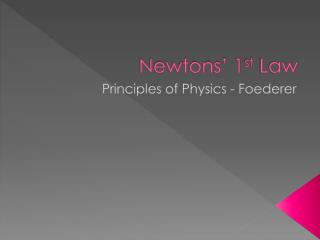DownloadDownload PresentationNewtons’ 1 st Law

# Newtons’ 1 st Law

Télécharger la présentation## Newtons’ 1 st Law

- - - - - - - - - - - - - - - - - - - - - - - - - - - E N D - - - - - - - - - - - - - - - - - - - - - - - - - - -
##### Presentation Transcript

1. Newtons’ 1st Law Principles of Physics - Foederer

2. Matter has has is has units of is a measure of Definition: is has units of Definition: Volume Mass Amount of space something occupies kg Inertia Resistance to changes in motion cm3, liters

3. Inertia • is a property of matter • is mass • is measured in kg • is not dependent on the motion of the object • As mass increases, inertia increases • As mass decreases, inertia decreases

4. Newton’s 1st Law Newton’s Laws help explain and predict the workings of the mechanical world. Newton’s 1st Law (N1L) – a.k.a. Law of Inertia A force is required to change the motion of an object

5. Newton’s 1st Law A change in motion could be: • speeding up • slowing down • stopping • starting • changing direction These are all examples of acceleration

6. Newton’s 1st Law • Objects will continue moving in straight paths at the same speed unless acted upon • In real world we don’t see this because of friction (a force that opposes motion) • Objects at rest will not move unless forced

7. According to N1L, a change in motion will only occur when a force causes it If no change in motion then either: 1. no forces are acting on it or 2. all forces cancel each other out (Fnet = 0)

8. F2 F1 When all forces cancel each other out Fnet = 0 Example Fnet = F1 – F2 = 0 **This object may be motionless or moving with constant speed (speed is unchanging).